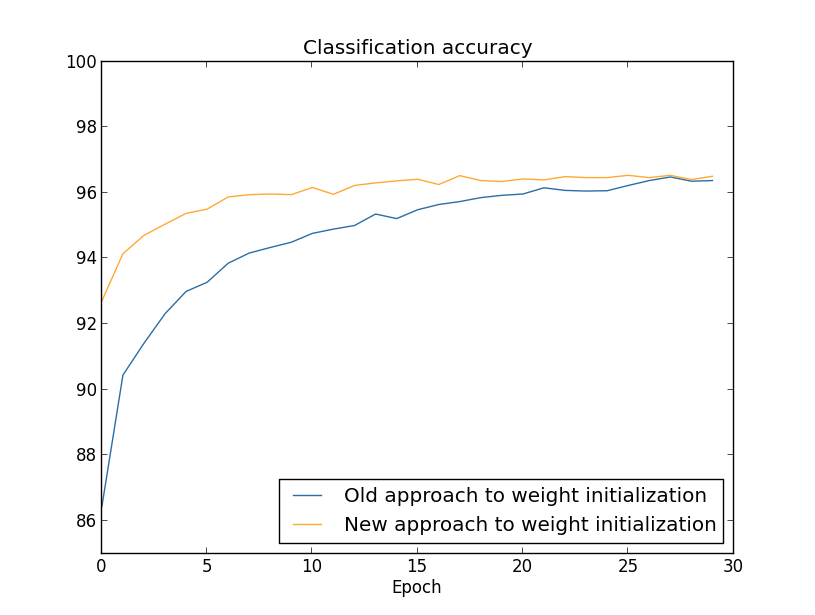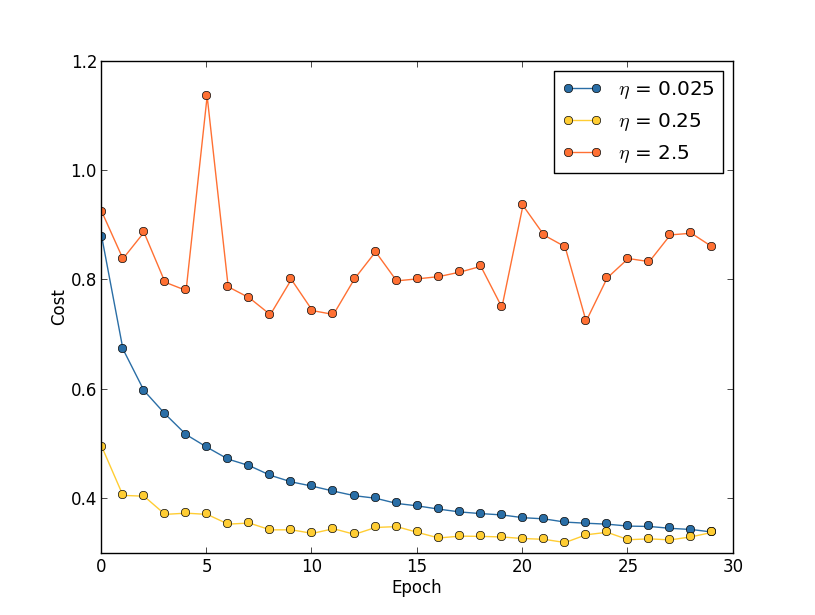# Neural Networks and Deep Learning（三·三）权重初始化及其他epoch

no-improvement-in-ten rule，就是说如果模型在最近的10个epoch中，验证集的accuracy都没有提高，则可以stop了。在早期实验中可以这么做，后续精细优化时可以改变ten，比如no-improvement-in-20/30等。

Mini-batch size

SGD优化的目标就是最小化损失函数$C$$C$是所有参数$w = w_1, w_2, \ldots$的函数，即$C=C(w)$。希望能够通过改变$w$，不断最小化$C$，即找一个$\Delta w$，使得$C(w+\Delta w)$最小化。把$C(w+\Delta w)$泰勒展开得到：

$\begin{eqnarray}C(w+\Delta w) & = & C(w) + \sum_j \frac{\partial C}{\partial w_j} \Delta w_j\nonumber \\ & & + \frac{1}{2} \sum_{jk} \Delta w_j \frac{\partial^2 C}{\partial w_j\partial w_k} \Delta w_k + \ldots\tag{1}\end{eqnarray}$

$\begin{eqnarray}C(w+\Delta w) = C(w) + \nabla C \cdot \Delta w +\frac{1}{2} \Delta w^T H \Delta w + \ldots,\tag{2}\end{eqnarray}$

$\begin{eqnarray} C(w+\Delta w) \approx C(w) + \nabla C \cdot \Delta w +\frac{1}{2} \Delta w^T H \Delta w.\tag{3}\end{eqnarray}$

$\begin{eqnarray}\Delta w = -H^{-1} \nabla C.\tag{4}\end{eqnarray}$$\begin{eqnarray} v & \rightarrow & v' = \mu v - \eta \nabla C \tag{5}\\w & \rightarrow & w' = w+v'.\tag{6}\end{eqnarray}$

tanh是和sigmoid很像的一个激活函数，其函数形式为：

$\begin{eqnarray}\tanh(z) \equiv \frac{e^z-e^{-z}}{e^z+e^{-z}}.\tag{7}\end{eqnarray}$

$\begin{eqnarray} \sigma(z) = \frac{1+\tanh(z/2)}{2},\tag{8}\end{eqnarray}$

$\tanh(z)$的函数图像如下，和sigmoid非常类似：$\tanh(z)$和sigmoid的主要区别就是值域不一样，前者值域为[-1,1]，后者值域为[0,1]。这会导致什么差异呢？观察(BP4)这个公式，对于第$l$层的第$j$个神经元和第$l-1$层的所有神经元的连接权重$w_{jk}^l$，如果使用sigmoid激活，则$a_k^{l-1}$都是非负的，而这些梯度共用一个$\delta_j^l$，所以对于固定的$j$，不同的$k$，所有的梯度$\frac{\partial C}{\partial w^l_{jk}}$方向是一样的！这在无形中就减小了搜索空间。而如果用$\tanh(z)$激活的话，不同的$k$$a^{l-1}_k$正负号可能就不一样，搜索空间更大，跟容易收敛。$ReLU(z)=max(0, z)\tag{9}$ReLU和Sigmoid、tanh很不一样，ReLU在$z>0$的方向上不会有梯度消失的问题。